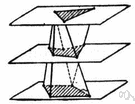# prismoid

Also found in: Thesaurus, Medical, Encyclopedia, Wikipedia.

## pris·moid

(prĭz′moid′)
n.
A prismatoid having polygons with the same number of sides as bases, and faces that are parallelograms or trapezoids.

[prism + -oid.]

## prismoid

(ˈprɪzmɔɪd)
n
(Mathematics) a prismatoid having an equal number of vertices in each of the two parallel planes and whose sides are trapeziums or parallelograms
[C18: from French prismoïde; see prism, -oid]

## pris•moid

(ˈprɪz mɔɪd)

n.
a solid having sides that are trapezoids and bases or ends that are parallel and similar but not congruent polygons. Compare prism (def. 2).
[1695–1705]
 Noun 1prismoid - a prismatoid whose bases are polygons having the same number of sides and whose other faces are trapezoids or parallelogramsprismatoid - a polyhedron whose vertices all lie in one or the other of two parallel planes; the faces that lie in those planes are the bases of the prismatoid